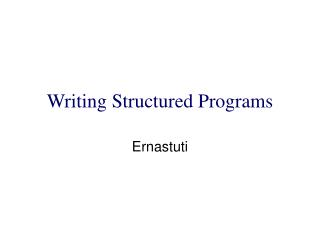DownloadDownload PresentationWriting Structured Programs

# Writing Structured Programs

Télécharger la présentation## Writing Structured Programs

- - - - - - - - - - - - - - - - - - - - - - - - - - - E N D - - - - - - - - - - - - - - - - - - - - - - - - - - -
##### Presentation Transcript

1. Writing Structured Programs Ernastuti

2. Four iteration statements 1. loop-repeat 2. while-repeat 3. loop-until-repeat 4. for-repeat

3. Loop-repeat loop read(x) if x= eof then exit endif call PROCESS(x) repeat S

4. While-repeat while conddo S repeat true cond S false

5. loop-until-repeat loop S until condrepeat false cond S true

6. For-repeat for vble start to finish by increment do S repeat false cond S true

7. for-repeat vble  start fin  finish incr  increment while ( vble – fin ) * incr 0do S vble  vble + incr repeat true cond S false for vble start to finish by increment do S repeat

8. The case for CASE if cond 1thenS1 elseifcond 2thenS2 elseifcond 3thenS3 . . elseifcond nthenSn elseSn+1 endif endif …endif case : cond 1: S1 : cond 2: S2 : cond n: Sn : else : Sn+1 endcase

9. Find the maximum of n numbers, n > 0 procedureMAX(A,n,j) // Set j so that A(j) is the maximum in A(1:n), n>0 // xmax  A(1); j  1 fori  2tondo IfA(i) >xmaxthen xmax  A(i); j i ; endif repeat endMAX

10. Find the maximum of n numbers, n > 0 procedureMAX1(i) //this is a function which returns the largest integer k such that A(k) is the maximum element in A(i:n)// globalintegern, A(1:n), j,k ; integeri if i < nthen jMAX1(i+1) if A(i) >A(j)thenk i elsek  j endif elsek  n endif return(k) endMAX1

11. procedureMAX2(i) local integerj,k;global integern, A(1:n); integeri integerSTACK(1:2*n); top  0 L1: ifi < n thentop  top + 1;STACK(top)  i top  top + 1; STACK(top)  2; i  i + 1 go to L1 L2:j  STACK(top); top top – 1 if A(i) >A(j)thenk i elsek  j endif elsek  n endif If top=0 then return(k) else addrSTACK(top); top top – 1 iSTACK(top); top top – 1 top top + 1; STACK(top)  k If addr = 2 then go toL2endif endif endMAX2

12. procedureMAX3(A,n) integer i,k,n; ik-n whilei>1do ii-1 if A(i) >A(k)thenkiendif repeat return(k) end MAX3

13. Read a set of values until their sum exceeds a predefined limit, say n y0 whiley  ndo read(x) yy+x repeat

14. Read in n values and process each one in some way i1 whilei  ndo read(x) call PROCESS(x) i  i + 1 repeat for i  1to ndo read(x) call PROCESS(x) repeat

15. Recursion The fibonacci sequence 1,1,2,3,5,8,13,21,34,… is defined as F0 = F1 = 1, Fi= Fi-1 + Fi-2 , i> 1 procedureF(n) //returns the nth Fibonacci number// Integern If n  1then return(1) else return(F(n-1) + F(n-2)) endif endF

16. procedure F1(n) // a function which returns the nth Fibonacci number// Ifn < 2then return(1) else return(F2(2,n,1,1)) endif endF1 procedureF2(i,n,x,y) Ifi  n then callF2(i+1,n,y,x+y) endif return(y) endF2

17. Computing the greatest common divisor of two nonnegative integers For example : gcd(22,8)= ? 22 = 1 x 2 x 11 8 = 1 x 23 gcd(22,8) = 2 Another way : gcd(22,8)=gcd(8,6)=gcd(6,2)=gcd(2,0)=2

18. Computing the greatest common divisor of two nonnegative integers For example : gcd(21,13)= ? 21 = 1 x 3 x 7 13 = 1 x 13  gcd(21,13) = 1 Another way : gcd(21,13)=gcd(13,8)=gcd(8,5)=gcd(5,3)= gcd(3,2)=gcd(2,1)=gcd(1,0)=1

19. Computing the greatest common divisor of two nonnegative integers procedure GCD(a,b) // assume a > b  0 // if b = 0then return(a) else return(GCD(b,a mod b)) endif endGCD

20. Computing the greatest common divisor of two nonnegative integers procedureGCD1(a,b) L1: ifb=0then return(a) elsetb;bamodb;a t; go to L1 endif endGCD1

21. Computing the greatest common divisor of two nonnegative integers procedureGCD2(a,b) whileb  0do tb;bamodb;a t repeat return(a) endGCD2

22. A procedure which searches for x in A(1:n) procedureSEARCH(i) //if there exists an index k such that A(k) = x in A(i:n), then the first such k is returned else zero is returned// globaln,x,A(1:n) case :i> n: return(0) :A(i) = x: return(i) : else : return(SEARCH(i+1)) endcase endSEARCH

23. Analyzing Algoriths x  x + y 1 for i 1 to n do x  x + y n repeat for i 1 to n do for i 1 to n do x  x + y n2 repeat repeat

24. Orders of magnitude • The order of magnitude of a statement refers to its frequency of execution while • The order of magnitude of an algorithm refers to the sum of the frequencies of all of its statements

25. Determining the order of magnitude of an algorithm is very important and producing an algorithm which is faster by an order of magnitude is a significant accomplishment. • The a priori analysis of algorithms is concerned chiefly with order of magnitude determination • Fortunately there is a convinient mathematical notation for dealing with this concept. • A priori = berdasarkan teori drpd kenyataan yg sebenarnya

26. Insertion Sort Insertion-Sort(A) cost times 1 forj 2to length[A] c1 n • dokey  A[j] c2 n-1 • Insert A[j] into the sorted sequence A[1..j-1] 0 n-1 4 i j -1 c4 n-1 • while i >0andA[i]>keyc5  tj • doA[i+1]A[i] c6  (tj – 1) 7 i i -1 c7  (tj – 1) 8 A[i+1] key c8 n-1

27. The running time of the algorithm is the sum of running time for each statement executed; • To compute (n), the running time of Insertion-Sort, we sum the products of the cost and times columns, obtaining (n) = c1n + c2(n-1) + c4(n-1)+ c5 tj + c6 (tj – 1) + c7 (tj – 1) + c8(n-1)